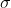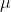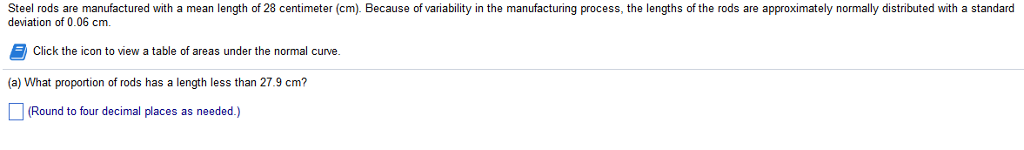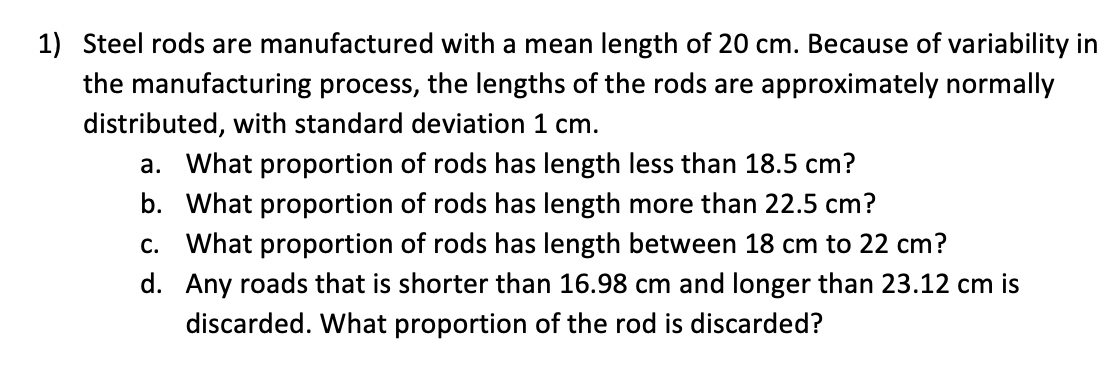Question

# Can you answer the following showing formula used and all workings out. The length of a...

Can you answer the following showing formula used and all workings out.

The length of a screw manufactured by a company is normally distributed with mean 2.5cm and a standard deviation 0.1cm. Specifications call for the lengths to range from 2.4cm to 2.6cm.

1, what value of standard deviation is required so that less than 7% of screws have a length greater than 2.8cm?

2, another manufacturer produces the same screw for which 15% of the screws have length less than 1.75cm and 12% of the screws have length higher than 3.2cm. Find the mean and standard deviation of the length of the screw manufactured by the company assuming the length is normally distributed.

1.

The mean length of the screw is 2.5 cm.

Letbe the value of standard deviation is required so that less than 7% of screws have a length greater than 2.8cm.

P(X > 2.8) = 0.07

P[Z > (2.8 - 2.5)/] = 0.07

0.3/= 1.476 (Using Standard normal distribution tables)= 0.3 / 1.476 = 0.2033 cm.

2.

Letandbe the length of the screw manufactured by the company.

Probability that screws have length less than 1.75cm = 0.15

P(X < 1.75) = 0.15

P[Z < (1.75 -) /] = 0.15

(1.75 -) /= -1.036 ---(1)     (Using Standard normal distribution tables)

Probability that screws have length higher than 3.2 cm = 0.12

P(X > 3.2) = 0.12

P[Z < (3.2 -) /] = 1.175

(3.2 -) /= 1.175 ---(2)    (Using Standard normal distribution tables)

Dividing (1) by (2), we get

(1.75 -) / (3.2 -) = -1.036 / 1.175

1.75 -= -0.8817 * (3.2 -)

1.75 -= -2.82144 + 0.88171.8817= 1.75 + 2.82144= 4.57144 / 1.8817 = 2.4294 cm

From (1),

(1.75 - 2.4294) /= -1.036= -0.6794 / -1.036 = 0.6558 cm

#### Earn Coins

Coins can be redeemed for fabulous gifts.

Similar Homework Help Questions
• ### Question 3: a.) A manufacturer knows that their items have a normally distributed length, with a...

Question 3: a.) A manufacturer knows that their items have a normally distributed length, with a mean of 19.6 inches, and standard deviation of 2.4 inches. If 4 items are chosen at random, what is the probability that their mean length is less than 17.5 inches? b.) A manufacturer knows that their items have a normally distributed lifespan, with a mean of 7.5 years, and standard deviation of 0.7 years. If you randomly purchase 14 items, what is the probability...

• ### A manufacturer knows that their items have a normally distributed length, with a mean of 18.8...

A manufacturer knows that their items have a normally distributed length, with a mean of 18.8 inches, and standard deviation of 1.5 inches. If 10 items are chosen at random, what is the probability that their mean length is less than 17.7 inches?

• ### A manufacturer knows that their items have a normally distributed length, with a mean of 18.8...

A manufacturer knows that their items have a normally distributed length, with a mean of 18.8 inches, and standard deviation of 1.5 inches. If 10 items are chosen at random, what is the probability that their mean length is less than 17.7 inches?

• ### 1) a) A manufacturer knows that their items have a normally distributed length, with a mean...

1) a) A manufacturer knows that their items have a normally distributed length, with a mean of 13.4 inches, and standard deviation of 2 inches. If one item is chosen at random, what is the probability that it is less than 7.5 inches long? b) A manufacturer knows that their items lifespans are normally distributed with mean = 14.2 and standard deviation = 3.9. What proportion of the items' lifespans will be longer than 25 years? c) A particular fruit's...

• ### A manufacturer knows that their items have a normally distributed length, with a mean of 18...

A manufacturer knows that their items have a normally distributed length, with a mean of 18 inches, and standard deviation of 5.7 inches. If one item is chosen at random, what is the probability that it is less than 13 inches long? A manufacturer knows that their items have a normally distributed lifespan, with a mean of 2.7 years, and standard deviation of 0.7 years. If you randomly purchase one item, what is the probability it will last longer than...

• ### A manufacturer knows that their items have a normally distributed length, with a mean of 18.1...A manufacturer knows that their items have a normally distributed length, with a mean of 18.1 inches, and standard deviation of 3.1 inches. If 5 items are chosen at random, what is the probability that their mean length is less than 20.7 inches? P(< 20.7) - Submit

• ### Steel rods are manufactured with a mean length of 28 centimeter (cm). Because of variability in...Steel rods are manufactured with a mean length of 28 centimeter (cm). Because of variability in the manufacturing process, the lengths of the rods are approximately normally distributed with a standard deviation of 0.06 cm. Click the icon to view a table of areas under the normal curve (a) What proportion o rods has a length less than 27.9 cm? Round to four decimal places as needed.)

• ### A manufacturer knows that their items have a normally distributed length, with a mean of 11.4...

A manufacturer knows that their items have a normally distributed length, with a mean of 11.4 inches, and standard deviation of 3.1 inches. If one item is chosen at random, what is the probability that it is less than 8.2 inches long?

• ### A manufacturer knows that their items have a normally distributed length, with a mean of 13.3...

A manufacturer knows that their items have a normally distributed length, with a mean of 13.3 inches, and standard deviation of 3.6 inches. If one item is chosen at random, what is the probability that it is less than 21.4 inches long?

• ### 1) Steel rods are manufactured with a mean length of 20 cm. Because of variability in...1) Steel rods are manufactured with a mean length of 20 cm. Because of variability in the manufacturing process, the lengths of the rods are approximately normally distributed, with standard deviation 1 cm. a. What proportion of rods has length less than 18.5 cm? b. What proportion of rods has length more than 22.5 cm? C. What proportion of rods has length between 18 cm to 22 cm? d. Any roads that is shorter than 16.98 cm and longer than...